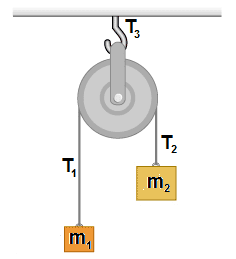# Can anybody check if I solved this correctly?(tension forces + pulley)

PhyIsOhSoHard

## Homework StatementThe pulley can rotate and has no friction.
Block $m_2$ is twice as big as $m_1$
Find the tensions, $T_1, T_2, T_3$

## Homework Equations

Newton's second law

## The Attempt at a Solution

Tension 1:
By creating a free body diagram only for the block, I got with Newton's second law:
$T_1-mg=ma$
$T_1=m(a+g)$

Tension 2:
$T_2-2mg=ma$
$T_2=m(a+2g)$

Tension 3:
$T_3-T_1-T_2=ma$

Inserting above values for the other tensions:
$T_3=ma+m(a+g)+m(a+2g)$
$T_3=3m(a+g)$

Did I solve this correctly? What about the acceleration? Can I do anything about that?

Last edited:

Enigman
$T_3-T_1-T_2=ma$

the pulley has no net force acting on it.

PhyIsOhSoHard
the pulley has no net force acting on it.

What exactly do you mean?
Are the 2 first tensions correct?

PhyIsOhSoHard
So we have:

$T_1-mg=ma$
$T_1=m(a+g)$

$2mg-T_2=ma$
$T_2=2m(g-a)$

Since the tension is uniform on the string:
$m(a+g)=2m(g-a)$
$a=\frac{1}{3}g$

Substituting this into the first equation:
$T_1-mg=\frac{1}{3}mg$
$T=\frac{4}{3}mg$

The tension of the pulley since it has no net force acting:
$T_3-T_1-T_2=0$

Since $T_1=T_2=T$
$T_3=2T$

Inserting the tensions:
$T_3=2\frac{4}{3}mg$

Last edited:
Homework Helper
Gold Member
So we have:

$T_1-mg=ma$
$T_1=m(a+g)$

$2mg-T_2=ma$
$T_2=2m(g-a)$

Since the tension is uniform on the string:
$m(a+g)=2m(g-a)$
$a=\frac{1}{3}g$

Substituting this into the first equation:
$T_1-mg=\frac{1}{3}mg$
$T=\frac{4}{3}mg$

The tension of the pulley since it has no net force acting:
$T_3-T_1-T_2=0$

Since $T_1=T_2=T$
$T_3=2T$

Inserting the tensions:
$T_3=2\frac{4}{3}mg$
Assuming the pulley has no moment of inertia, that all looks correct. I wouldn't write the final answer as $T_3=2\frac{4}{3}mg$ though. It looks like you mean "two and four thirds".